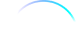## FANDOM

49,098 Pages

The Drake equation was devised in an attempt to estimate the number of extraterrestrial alien species that could communicate with Humans. The first and more mathematical variation stated:

$N = R^{*} \times f_{p} \times n_{e} \times f_{l} \times f_{i} \times f_{c} \times L$
where:
• N is the number of extraterrestrial civilizations in our galaxy with which we might expect to be able to communicate
and
• R* is the rate of star formation in our galaxy
• fp is the fraction of those stars which have planets
• ne is average number of planets which can potentially support life per star that has planets
• fl is the fraction of the above which actually go on to develop life
• fi is the fraction of the above which actually go on to develop intelligent life
• fc is the fraction of the above which are willing and able to communicate
• L is the expected lifetime of such a civilization

The second variation stated:

$F f ^ 2 (M g E) - C ^ 1 Ri^1 \times M = L / So$

In 1996, Rain Robinson had a poster of the two variations of the equation right behind her computer monitor in her office at the Griffith Observatory. (VOY: "Future's End")

The Drake equation was devised by Dr. Frank Drake in 1961. It was used by Gene Roddenberry in 1964 in his pitch for Star Trek. He did not have a copy of the equation so he made up his own, which is the "second variation" from "Future's End". According to the Star Trek Encyclopedia (4th ed., vol. 1, p. 218), Drake himself visited the set one day, and even viewed Roddenberry's "second variation", before gently pointing out that a value raised to the first power is merely the value itself.

## External linkEdit

Community content is available under CC-BY-NC unless otherwise noted.